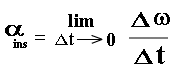# Angular Velocity-Angular Acceleration

Definition
Angle traversed by a body in circular motion in unit time is called angular velocity. Or,
The rate of change of angular position of a body is called angular velocity.
Consider a particle “P” moving in a circular path of radius “r”. The angular position P1 is q1 at instant t1 and q2 at instant t2.
The angular displacement is q2 – q1 = Dq .
Time interval = t2 –t1 =Dt
Since the angle traced per sec by a body moving in circle is called its average angular speed
denoted by wav.
Therefore, wav = Dq / DtUnit for angular velocity is rad /sec OR rev/sec.

Instantaneous angular velocity
The angular velocity at a particular instant is called instantaneous angular velocity. If D t is very small i.e. D t è 0 then Dq / Dt is called instantaneous angular velocity.
Unit for angular velocity is rad /sec OR rev/sec.

Direction of angular velocity
Direction of angular velocity is given by right-hand rule, which states that if we curl the fingers of right hand in the direction of rotation then thumb will represent the direction of angular velocity.

Angular acceleration
Rate of change of angular velocity of a body is called angular acceleration. It is denoted by a.
If w1 is angular velocity of a body moving in circle at instant t1 and w2 is angular velocity of body at
instant t2 then,If Dt is very small then acceleration is called instantaneous angular acceleration.Unit
Unit of angular acceleration is rad/sec2.

Direction
Direction of angular acceleration is same as of w.